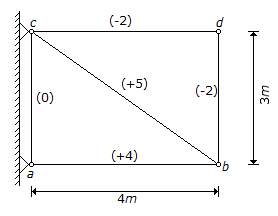# Civil Engineering - GATE Exam Questions

### Exercise :: GATE Exam Questions - Section 6

46.

For a highway with design speed of 100 kmph, the safe overtaking sight distance is (assume accelerations as 0.53 m/sec2)

 A. 300 m B. 750 m C. 320 m D. 470 m

Explanation:

No answer description available for this question. Let us discuss.

47.

For a flow of 5.7 MLD (million litres per day) and a detention time of 2 hours, the surface area of a rectangular sedimentation tank to remove all particles having settling velocity of 0.33 mm/s is

 A. 20 m2 B. 100 m2 C. 200 m2 D. 400 m2

Explanation:

No answer description available for this question. Let us discuss.

48.

If the deformations of the truss-members are as shown in parentheses, the rotation of the member bd is :Explanation:

No answer description available for this question. Let us discuss.

49.

The shape of clay particle is usually

 A. angular B. flaky C. tubular D. rounde

Explanation:

No answer description available for this question. Let us discuss.

50.

As per IS : 800-1984 the maximum allowable slenderness ratio of compression members carrying forces resulting from dead load and superimposed load is

 A. 180 B. 250 C. 300 D. 400